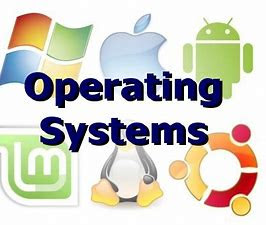# C- program of look disk scheduling in operating system

What is Look disk scheduling:-

It is similar to the SCAN disk scheduling algorithm except for the difference that the disk arm in spite of going to the end of the disk goes only to the last request to be serviced in front of the head and then reverses its direction from there only. Thus it prevents the extra delay which occurred due to unnecessary traversal to the end of the disk.

1. If there are no requests left to be services the Head will not move to the end of the disk unlike SCAN algorithm.
2. Low variance is provided in waiting time and response time

1. Overhead of finding the end requests is present.

Code:-

#include<stdio.h>
#include<stdlib.h>
int main()
{
printf(“Enter the number of Requestsn”);
scanf(“%d”,&n);
printf(“Enter the Requests sequencen”);
for(i=0;i<n;i++)
scanf(“%d”,&RQ[i]);
printf(“Enter initial head positionn”);
scanf(“%d”,&initial);
printf(“Enter total disk sizen”);
scanf(“%d”,&size);
printf(“Enter the head movement direction for high 1 and for low 0n”);
scanf(“%d”,&move);
// logic for look disk scheduling
/*logic for sort the request array */
for(i=0;i<n;i++)
{
for(j=0;j<ni1;j++)
{
if(RQ[j]>RQ[j+1])
{
int temp;
temp=RQ[j];
RQ[j]=RQ[j+1];
RQ[j+1]=temp;
}

}
}

int index;
for(i=0;i<n;i++)
{
if(initial<RQ[i])
{
index=i;
break;
}
}
// if movement is towards high value
if(move==1)
{
for(i=index;i<n;i++)
{
initial=RQ[i];
}
for(i=index1;i>=0;i–)
{
initial=RQ[i];
}
}
// if movement is towards low value
else
{
for(i=index1;i>=0;i–)
{
initial=RQ[i];
}
for(i=index;i<n;i++)
{
initial=RQ[i];
}
}
return 0;
}

Output:-

Enter the number of Request
8
Enter the Requests Sequence
95 180 34 119 11 123 62 64
Enter initial head position
50
Enter the head movement direction for high 1 and for low 0
1
Total head movement is 299Must visit:-

Recommended Post:

Hackerearth Problems:-

Data structure:-

Key points:-

MCQs:-